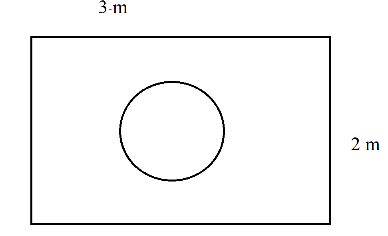# Ex.15.1 Q20 Probability Solution - NCERT Maths Class 10

Go back to  'Ex.15.1'

## Question

Suppose you drop a die at random on the rectangular region shown in figure. What is the probability that it will land inside the circle with diameter $$1\rm{m}$$?Video Solution
Probability
Ex 15.1 | Question 20

## Text Solution

What is known?

A die is dropped at random on the rectangular region as shown in figure.

Length of rectangular region $$=3\,\rm{m}$$

Breadth of rectangular region $$=2\,\rm{m}$$

Diameter of the circle $$=1 \,\rm{m}$$

∴ Radius of the circle $$=0.5\,\rm{m}$$

What is unknown?

The probability that the die dropped at random will land inside the circle with diameter $$1\,\rm{m}$$?

Steps:

Area of rectangular region

\begin{align}\text{}& =L\,\times B \\ {} & =3\,\times 2 \\{} & =6{{\text{m}}^{2}} \\\end{align}

Diameter of circular region $$=1 \rm{m}$$ 

Radius of circular region \begin{align}=\frac{1}{2}\rm{m}\end{align}

Area of circular region

\begin{align} &= \pi r^{2} \\ & =\pi \,\times {{\left( \frac{1}{2} \right)}^{2}} \\ &=\frac{\pi }{4}\end{align}

Probability that it will land inside the circle

\begin{align}& =\frac{\begin{bmatrix} \text { Number of}\\ \text{ possible outcomes }\end{bmatrix} }{ \begin{bmatrix}\text { Total no of} \\ \text{favorable outcomes} \end{bmatrix} } \\ & =\quad \frac{\text{Area of circular region}}{\text{Area of rectangular region}} \\ & =\quad \frac{\frac{\pi }{4}}{6} \\ & =\frac{\pi }{24} \end{align}

The probability that it will land inside the circle is \begin{align}\frac{\pi}{24}\end{align}

Learn from the best math teachers and top your exams

• Live one on one classroom and doubt clearing
• Practice worksheets in and after class for conceptual clarity
• Personalized curriculum to keep up with school